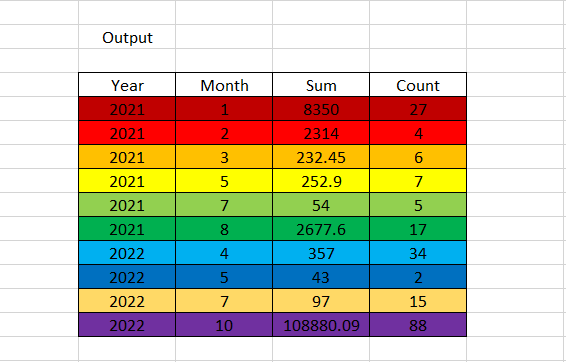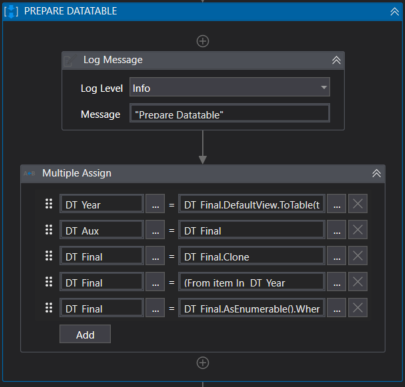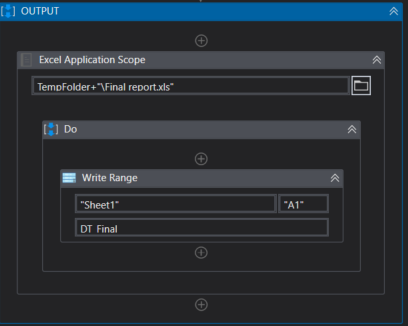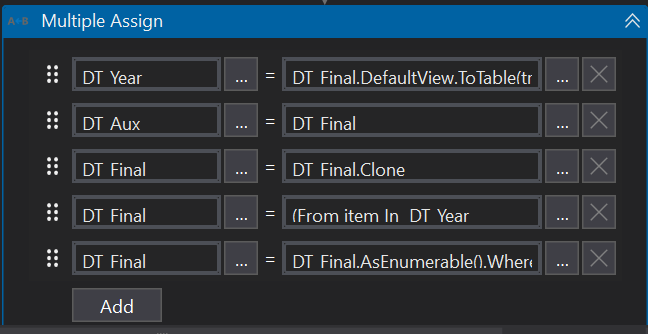# Add multiple datatable in for each loop

I have these two datatables. String dt1_1 and dt_2 is a part of the datatable.

And I would like this two to be added to become like this (color as a guide).The input datatable is limitless. It can be only 1 or 3 or 12. Each datatable is in for each loop. dt_1 is in the first loop and dt_2 is in the second loop.

Hi @arina ,

Do you need to read the excels to extract the information to create the output?

Because, you can follow these steps:

1. Create a local folder where the excels are to extract the information according to the number of entries (It will be dynamic)

2. Create a variable where you will store the number of entries to create a for each.

3. Extract information with a temporary datatable to merge a information with the final datatable.

4. With LINQ combine Month, Sum and Count according to the year and month.

If you need the example, let me know.
Have a great day!

Hey @arina ,

Is all the table would be having the same number of columns and exists in separate sheets?

Thanks,
Sanjit

The number of column is consistent. And each datatable exist in different workbook.

Yes, I’ll need to read excel to extract information.

May I have an example? I manage to do up until 3 (partially)

@arina

1. Read all the excel and merge them into one datatable.
2. using linq you can get the required sum and count as per year and month.

Linq to get the sum and count

(From table In dt1.AsEnumerable
Where Not table(“Year”).ToString.Contains(“Total”)
Group table By Year = table(“Year”).ToString, Month = table(“Month”).ToString Into grp = Group
Let sum1 = grp.Sum(Function (x) CDbl (x(“Sum”).toString.Trim))
Let Count1 = grp.Sum(Function (x) CDbl(x(“Count”).toString.Trim))
Select dtFinal.Rows.Add({Year, Month, sum1, Count1 })
).CopyToDataTable

Attaching the example with two datatables here
ExcelSUm.xaml (10.8 KB)

Hope this will help youHi @arina,

Of course, I have attached an example where the explanation is below:

1. Read Excels: It reads the excels in a local folder to merge them to DT_Final.

2. Prepare datatable: It combines Sum and Count according to the year and month with LINQ and removes lines where appears Total.3. Output: It show us the result.Example:
Multiple Datatable.zip (4.3 KB)

Have a great day!
Please let me know if you need anything else.

@Pamela_Sibaja I followed your method for the merge, but I currently have 1 input datatable only. Somehow the value duplicated two times. But it shouldn’t since I only have 1 input datatable. dt_Temp is an empty datatable just like your dt_Final.

And I did this linq by @SaurabhDisawal and the table is duplicated for another two times.

For example. Year 2021, Month 6, Sum 100, Count 2 become Year 2021, Month 6, Sum 200, Count 4 outside the for each loop. Then it became Year 2021, Month 6, Sum 400, Count 800 after linq.

Could you please send me the data extracted of the datatable named Out_dt_Pivot?
It’s to review the information.

Here’s it
input.xlsx (1.6 MB)

@arina

If you only have one input then no need to do all this right, you need to merge the tables only when you have more than one input datatable.

code sequence should be followed like this

1. if you have only one input then no need to merge
2. more than one input, merge dt1 with dt2 in dt1 and then dt1 with dt3 in dt1 and so on and then you have to use the linq to get the desired result.

Hi @arina,

You can use an if activity to validate if there are many files in the folder that you can merge, but if it’s just one you can continue with my example code and it will work fine.

Have a great day!
Please let me know if you need anything else.

Example:
Multiple Datatable.zip (4.5 KB)

I have a problem on the fourth expression when I changed it to my own variable

Hi @arina ,
Where you wrote Let out_dt_Pivot should be Let DT_Temp, because I’m creating a new datatable where I pull the Unique values ​​from the datatable to compare DT_Aux values ​​and combine lines and add equal values ​​based on Year and Month column in DT_Temp to add to DT_Final which for you would be out_dt_Pivot.

Also, where you see DT_Final, you should change to out_dt_Pivot.
Because I think out_dt_Pivot has all data.you can merge
dtOne.Merge(dtTwo);

This topic was automatically closed 3 days after the last reply. New replies are no longer allowed.